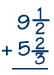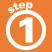Home    |    Teacher    |    Parents    |    Glossary    |    About UsThe steps are the same whether you're adding or subtracting mixed numbers:

1. Find the Least Common Denominator (LCD)
2. Find the equivalent fractions.
3. Add or subtract the fractions and add or subtract the whole numbers.
 Homework Help | Pre-Algebra | FractionsEmail this page to a friendSearch·  Definitions·  Reducing fractions·  Adding and subtract-     ing fractions·  Multiplying fractions·  Dividing fractions·  Adding and subtract-     ing mixed numbers·  Multiplying     mixed numbers·  Dividing     mixed numbersFirst Glance In Depth Examples WorkoutAdding and subtracting mixed numbers